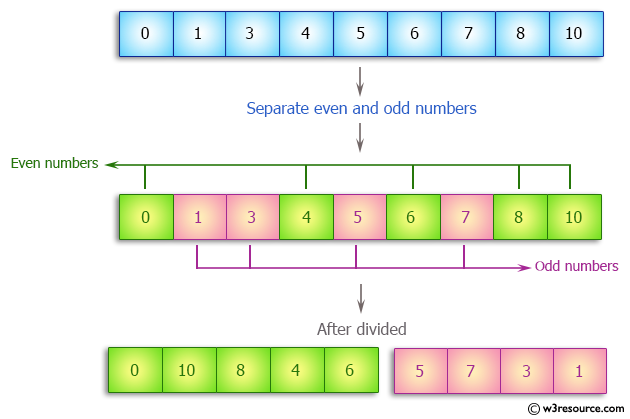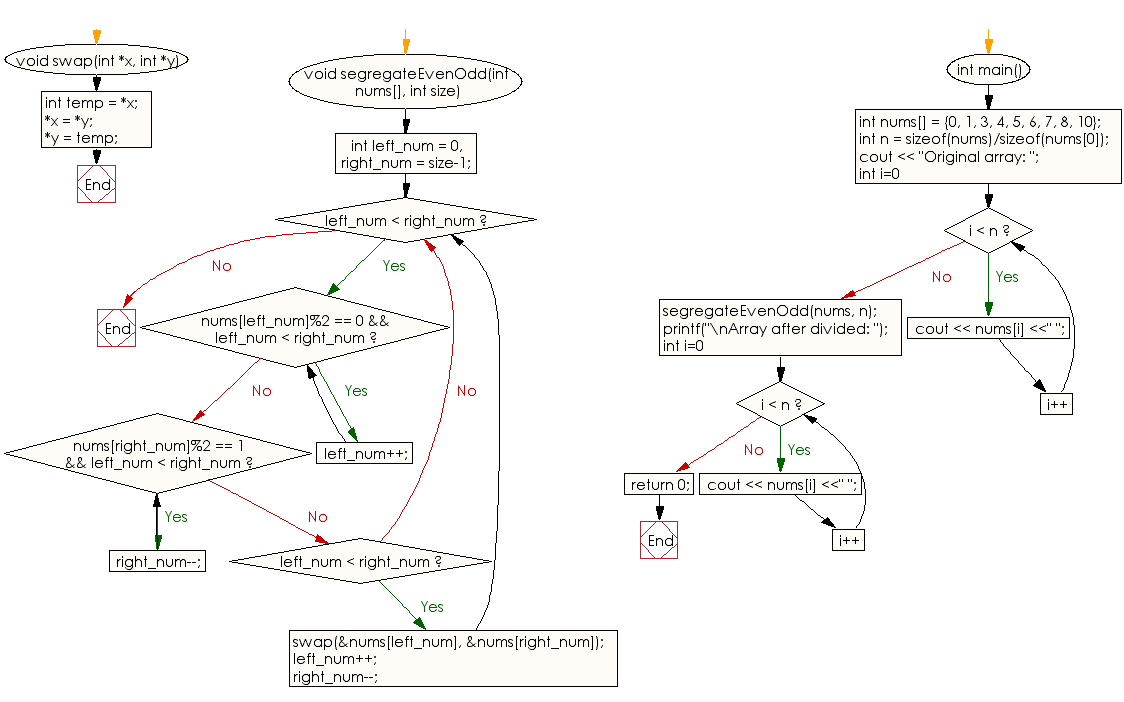﻿ C++ : Separate even and odd numbers of an array of integers# C++ Exercises: Separate even and odd numbers of an array of integers. Put all even numbers first, and then odd numbers

## C++ Array: Exercise-13 with Solution

Write a C++ program to separate even and odd numbers of an array of integers. Put all even numbers first, and then odd numbers.

Pictorial Presentation:Sample Solution:

C++ Code :

``````#include<iostream>
using namespace std;

void swap(int *x, int *y)
{
int temp = *x;
*x = *y;
*y = temp;
}

void segregateEvenOdd(int nums[], int size)
{
int left_num = 0, right_num = size-1;
while (left_num < right_num)
{
while (nums[left_num]%2 == 0 && left_num < right_num)
left_num++;

while (nums[right_num]%2 == 1 && left_num < right_num)
right_num--;

if (left_num < right_num)
{
swap(&nums[left_num], &nums[right_num]);
left_num++;
right_num--;
}
}
}

int main()
{
int nums[] = {0, 1, 3, 4, 5, 6, 7, 8, 10};
int n = sizeof(nums)/sizeof(nums);
cout << "Original array: ";
for (int i=0; i < n; i++)
cout << nums[i] <<" ";
segregateEvenOdd(nums, n);

printf("\nArray after divided: ");
for (int i=0; i < n; i++)
cout << nums[i] <<" ";
return 0;
}
``````

Sample Output:

```Original array: 0 1 3 4 5 6 7 8 10
Array after divided: 0 10 8 4 6 5 7 3 1
```

Flowchart:C++ Code Editor:

What is the difficulty level of this exercise?

Test your Programming skills with w3resource's quiz.

﻿